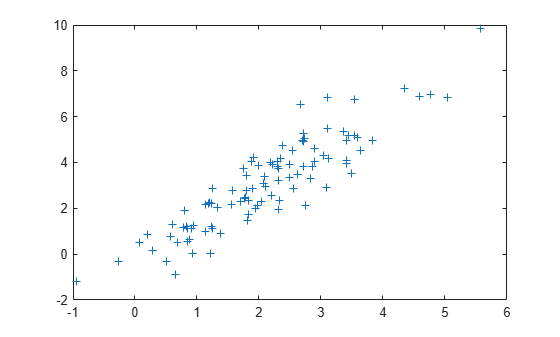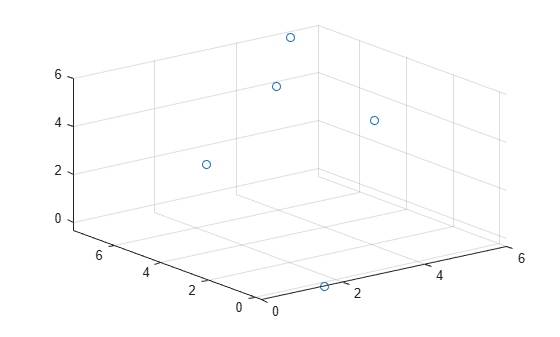# mvnrnd

Multivariate normal random numbers

## Syntax

``R = mvnrnd(mu,Sigma,n)``
``R = mvnrnd(mu,Sigma)``

## Description

example

````R = mvnrnd(mu,Sigma,n)` returns a matrix `R` of `n` random vectors chosen from the same multivariate normal distribution, with mean vector `mu` and covariance matrix `Sigma`. For more information, see Multivariate Normal Distribution. ```

example

````R = mvnrnd(mu,Sigma)` returns an m-by-d matrix `R` of random vectors sampled from m separate d-dimensional multivariate normal distributions, with means and covariances specified by `mu` and `Sigma`, respectively. Each row of `R` is a single multivariate normal random vector.```

## Examples

collapse all

Generate random numbers from the same multivariate normal distribution.

Define `mu` and `Sigma`, and generate 100 random numbers.

```mu = [2 3]; Sigma = [1 1.5; 1.5 3]; rng('default') % For reproducibility R = mvnrnd(mu,Sigma,100);```

Plot the random numbers.

`plot(R(:,1),R(:,2),'+')`Randomly sample from five different three-dimensional normal distributions.

Specify the means `mu` and the covariances `Sigma` of the distributions. Let all the distributions share the same covariance matrix, but vary the mean vectors.

```firstDim = (1:5)'; mu = repmat(firstDim,1,3)```
```mu = 5×3 1 1 1 2 2 2 3 3 3 4 4 4 5 5 5 ```
`Sigma = eye(3)`
```Sigma = 3×3 1 0 0 0 1 0 0 0 1 ```

Randomly sample once from each of the five distributions.

```rng('default') % For reproducibility R = mvnrnd(mu,Sigma)```
```R = 5×3 1.5377 -0.3077 -0.3499 3.8339 1.5664 5.0349 0.7412 3.3426 3.7254 4.8622 7.5784 3.9369 5.3188 7.7694 5.7147 ```

Plot the results.

`scatter3(R(:,1),R(:,2),R(:,3))`## Input Arguments

collapse all

Means of multivariate normal distributions, specified as a `1`-by-d numeric vector or an m-by-d numeric matrix.

• If `mu` is a vector, then `mvnrnd` replicates the vector to match the trailing dimension of `Sigma`.

• If `mu` is a matrix, then each row of `mu` is the mean vector of a single multivariate normal distribution.

Data Types: `single` | `double`

Covariances of multivariate normal distributions, specified as a d-by-d symmetric, positive semi-definite matrix or a d-by-d-by-m numeric array.

• If `Sigma` is a matrix, then `mvnrnd` replicates the matrix to match the number of rows in `mu`.

• If `Sigma` is an array, then each page of `Sigma`, `Sigma(:,:,i)`, is the covariance matrix of a single multivariate normal distribution and, therefore, is a symmetric, positive semi-definite matrix.

If the covariance matrices are diagonal, containing variances along the diagonal and zero covariances off it, then you can also specify `Sigma` as a `1`-by-d vector or a `1`-by-d-by-m array containing just the diagonal entries.

Data Types: `single` | `double`

Number of multivariate random numbers, specified as a positive scalar integer. `n` specifies the number of rows in `R`.

Data Types: `single` | `double`

## Output Arguments

collapse all

Multivariate normal random numbers, returned as one of the following:

• m-by-d numeric matrix, where m and d are the dimensions specified by `mu` and `Sigma`

• `n`-by-d numeric matrix, where `n` is the specified input argument and d is the dimension specified by `mu` and `Sigma`

If `mu` is a matrix and `Sigma` is an array, then `mvnrnd` computes `R(i,:)` using `mu(i,:)` and `Sigma(:,:,i)`.

collapse all

### Multivariate Normal Distribution

The multivariate normal distribution is a generalization of the univariate normal distribution to two or more variables. It has two parameters, a mean vector μ and a covariance matrix Σ, that are analogous to the mean and variance parameters of a univariate normal distribution. The diagonal elements of Σ contain the variances for each variable, and the off-diagonal elements of Σ contain the covariances between variables.

The probability density function (pdf) of the d-dimensional multivariate normal distribution is

where x and μ are 1-by-d vectors and Σ is a d-by-d symmetric, positive definite matrix. Only `mvnrnd` allows positive semi-definite Σ matrices, which can be singular. The pdf cannot have the same form when Σ is singular.

The multivariate normal cumulative distribution function (cdf) evaluated at x is the probability that a random vector v, distributed as multivariate normal, lies within the semi-infinite rectangle with upper limits defined by x:

`$\mathrm{Pr}\left\{v\left(1\right)\le x\left(1\right),v\left(2\right)\le x\left(2\right),...,v\left(d\right)\le x\left(d\right)\right\}.$`

Although the multivariate normal cdf does not have a closed form, `mvncdf` can compute cdf values numerically.

## Tips

• `mvnrnd` requires the matrix `Sigma` to be symmetric. If `Sigma` has only minor asymmetry, you can use `(Sigma + Sigma')/2` instead to resolve the asymmetry.

• In the one-dimensional case, `Sigma` is the variance, not the standard deviation. For example, `mvnrnd(0,4)` is the same as `normrnd(0,2)`, where `4` is the variance and `2` is the standard deviation.

 Kotz, S., N. Balakrishnan, and N. L. Johnson. Continuous Multivariate Distributions: Volume 1: Models and Applications. 2nd ed. New York: John Wiley & Sons, Inc., 2000.